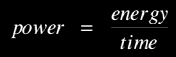# The kilo-watt-hour (kWh)

First things first. The kilowatt-hour (kWh) is not a unit of power. It is a unit of energy. Here's why:

The kW is a unit of power. It is 1000 W or 1000 J/s.

By definition:Rearranging this we get energy = power × time

If we choose to measure power in kW and time in hours, we have:

energy (kWh) = power (kW) × time (h)Energy MenuElectricity MenuGo to next page